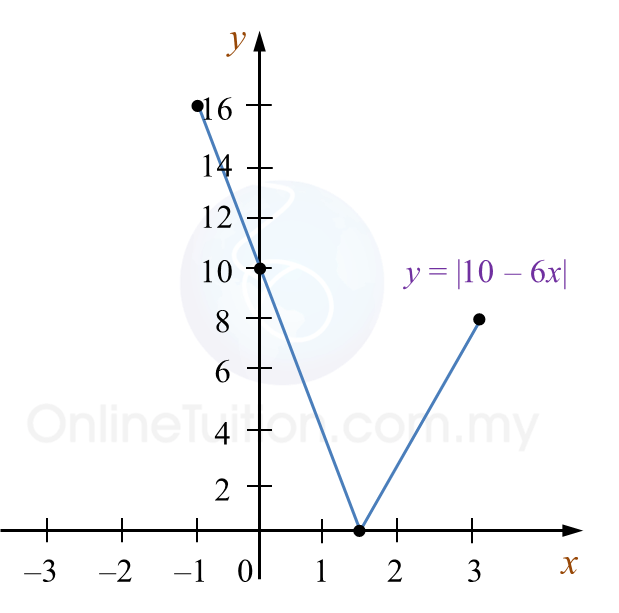# SPM Additional Mathematics 2018, Paper 2 (Question 1 & 2)

Question 1 (6 marks):
The sum of the first n terms of an arithmetic progression, Sn is given by ${S}_{n}=\frac{3n\left(n-33\right)}{2}.$
Find
(a) the sum of the first 10 terms,
(b) the first term and the common difference,
(c) the value of q, given that qth term is the first positive term of the progression.

Solution:
(a)
$\begin{array}{l}{S}_{n}=\frac{3n\left(n-33\right)}{2}\\ {S}_{10}=\frac{3\left(10\right)\left(10-33\right)}{2}\\ {S}_{10}=-345\end{array}$

(b)

(c)

Question 2 (8 marks):
It is given that g : x → 2x – 3 and h : x → 1 – 3x.
(a) Find
(i) h (5)
(ii) the value of k if $g\left(k+2\right)=\frac{1}{7}h\left(5\right),$
(iii) hg(x).

(b)
Hence, sketch the graph of y = | hg(x) | for –1 ≤ x ≤ 3.
State the range of y.

Solution:
(a)(i)

(a)(ii)
$\begin{array}{l}g\left(x\right)=2x-3\\ g\left(k+2\right)=\frac{1}{7}h\left(5\right)\\ 2\left(k+2\right)-3=\frac{1}{7}\left(-14\right)\\ 2k+4-3=-2\\ 2k=-3\\ k=-\frac{3}{2}\end{array}$

(a)(iii)

(b)
y = |hg(x)|,
y = |10 – 6x|
Range of y : 0 ≤ y ≤ 16